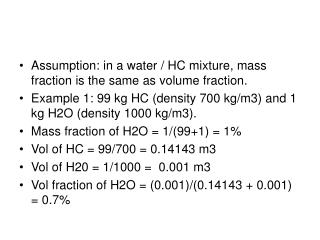DownloadDownload PresentationAssumption: in a water / HC mixture, mass fraction is the same as volume fraction.

# Assumption: in a water / HC mixture, mass fraction is the same as volume fraction.

Télécharger la présentation## Assumption: in a water / HC mixture, mass fraction is the same as volume fraction.

- - - - - - - - - - - - - - - - - - - - - - - - - - - E N D - - - - - - - - - - - - - - - - - - - - - - - - - - -
##### Presentation Transcript

1. Assumption: in a water / HC mixture, mass fraction is the same as volume fraction. • Example 1: 99 kg HC (density 700 kg/m3) and 1 kg H2O (density 1000 kg/m3). • Mass fraction of H2O = 1/(99+1) = 1% • Vol of HC = 99/700 = 0.14143 m3 • Vol of H20 = 1/1000 = 0.001 m3 • Vol fraction of H2O = (0.001)/(0.14143 + 0.001) = 0.7%

2. Example 2: 70 kg HC (density 700 kg/m3) and 30 kg H2O (density 1000 kg/m3). • Mass fraction of H2O = 30/(70+30) = 30% • Vol of HC = 70/700 = 0.1 m3 • Vol of H20 = 30/1000 = 0.03 m3 • Vol fraction of H2O = (0.03)/(0.1 + 0.03) = 23% • Assumption mass and volume fraction are the same is wrong.